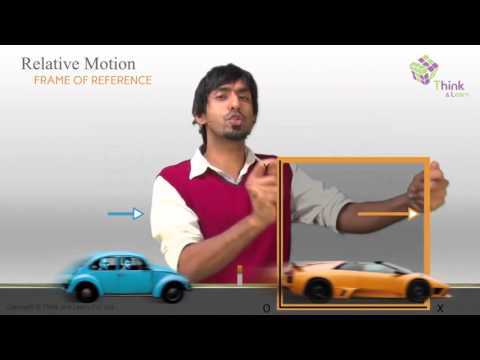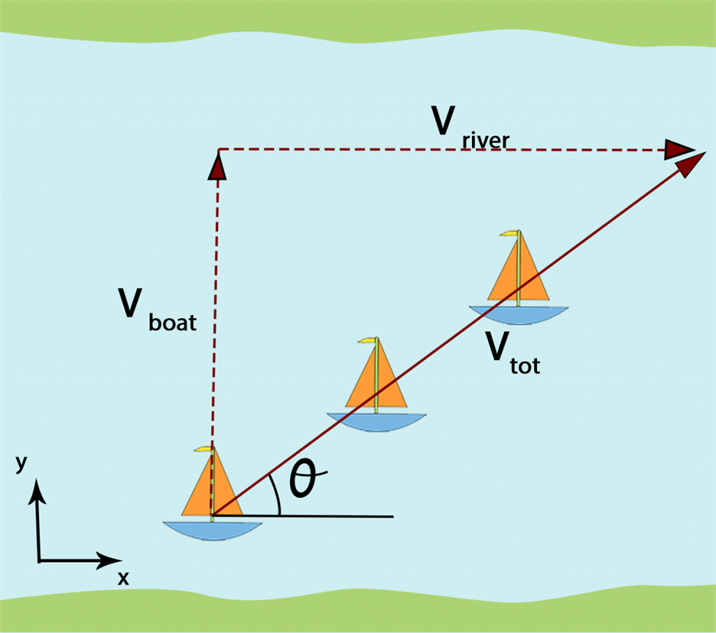# Relative Motion

The relative velocity is the velocity of an object A or observer A in the rest frame of (or with respect to) another object B or observer B. This is important because the observed motion may appear different from one frame of reference to another. The best example is, when you travel by train, you feel trees are moving back.## What is Relative Motion?

Relative motion is basically observing the motion of an object from another object. For example, a person standing on the ground is at rest with respect to the ground but he will be in motion with respect to the moon. Thus, it is evident that the observed motion depends on the frames of reference.

## Relative Motion Formula

The formula for relative velocity as per relative motion is –

 Va,b = Va,g − Vb,g

Where,

• Va,b = Velocity of a relative to b or the resultant velocity
• Va,g = Velocity of a relative to the ground
• Vb,g = Velocity of b relative to the ground

## Relative Motion Examples

Sitting on your chair? How fast are you are moving?

• Relative to Ground: Zero
You are not at all moving with respect to ground (Considering ground as the frame of reference)
• Relative to the Sun: 2.97×104 m/s
From Kepler’s laws of planetary motion, we know that the earth is orbiting around the sun at the speed of 2.97×104 m/s. Although you are sitting on your chair, an observer on the Sun would see you moving with the speed of 2.97×104 m/s.

Both the answers are correct with respect to the frames of reference considered.

## Relative Motion Problems

Example 1:

Now suppose a river is flowing with a velocity of 4 km/h. So, how much time a man will take to cross the river if there is no drift between the start and finishing point. The velocity of the man is 5 km/h and the width of the river is 600 m.

Solution:Let the velocity of the man be x î + y ĵ with respect to the river.

Vm = Vm,r + VrVm
= (x+4) î + y ĵ

As observed from the ground the velocity along the x-direction is zero (Since the drift is zero).

So, x = −4km/h —– (1)

Also, x2 + y2 = 25 —– (2)

Using (1) and (2), we get:
y = 3 km/h
Vm = 3 ĵ

Now considering motion perpendicular to the flow of river, s = 0.6 km v = 3 km/h
Therefore, time taken to cross the river is, t= 0.2 h.

Example 2:
Another example of relative motion is a man holding an umbrella to negotiate rain. When he is standing, he holds the umbrella at an angle of 30° with the vertical. When he starts running at 10 km/h the rain appears to fall vertically. We need to find the velocity of rain.

Solution:
Let the relative motion or relative velocity of rain with respect to ground be x î + y ĵ. Vr,m = (x–10) î + y ĵ

Since the rain appears to fall vertically so the <a href=”https://byjus.com/physics/horizontal-motion/”> horizontal motion </a> component will be zero.

Hence, x = 10 km/h —- (1)

Also, the rain makes an angle 30° with the vertical so, tan30° = xy —– (2)

Using (1) and (2) we get, y = 10√3 km/h

So the velocity of the rain is, Vr = 10î + 10√3ĵ

Example 3:

If a car A goes from left to right with a velocity of 20 m/s and car B goes from right to left with a speed of 30 km per hour. What is the velocity of car B with respect to car A?

Solution: The formula for relative velocity is Va,b = Va − Vb Thus, substituting the values we get 20-(-30)=50m/s.

Example 4:

If a car A goes from left to right with a velocity of 20 m/s and car B goes from left to right with a speed of 30 km per hour. What is the velocity of car B with respect to car A?

Solution: The formula for relative velocity is Va,b = Va − Vb Thus, substituting the values we get 20-(30)=|-10|m/s = 10m/s.

Example 5:

If person A is sitting inside a bus, which is moving at the speed of 10m/s.
(i) What is the relative speed of person A with respect to the bus?
(ii) What is the relative speed of person A with respect to the ground?

Solution: (i) The relative speed of person A with respect to the bus is zero m/s.
(ii) The relative speed of person A with respect to the ground is 10m/s.